• 问答
• 技术
• 实践
• 资源
"未来"的经典之作 ViT：transformer is all you need!

ViT（vision transformer）是Google在2020年提出的直接将transformer应用在图像分类的模型，后面很多的工作都是基于ViT进行改进的。ViT的思路很简单：直接把图像分成固定大小的patchs，然后通过线性变换得到patch embedding，这就类比NLP的words和word embedding，由于transformer的输入就是a sequence of token embeddings，所以将图像的patch embeddings送入transformer后就能够进行特征提取从而分类了。ViT模型原理如下图所示，其实ViT模型只是用了transformer的Encoder来提取特征（原始的transformer还有decoder部分，用于实现sequence to sequence，比如机器翻译）。下面将分别对各个部分做详细的介绍。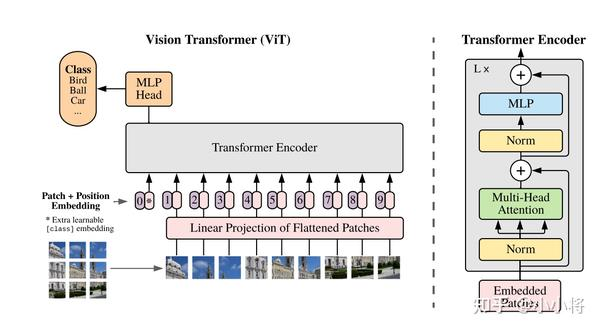## Patch Embedding

class PatchEmbed(nn.Module):
""" Image to Patch Embedding
"""
def __init__(self, img_size=224, patch_size=16, in_chans=3, embed_dim=768):
super().__init__()
img_size = to_2tuple(img_size)
patch_size = to_2tuple(patch_size)
num_patches = (img_size // patch_size) * (img_size // patch_size)
self.img_size = img_size
self.patch_size = patch_size
self.num_patches = num_patches

self.proj = nn.Conv2d(in_chans, embed_dim, kernel_size=patch_size, stride=patch_size)

def forward(self, x):
B, C, H, W = x.shape
# FIXME look at relaxing size constraints
assert H == self.img_size and W == self.img_size, \
f"Input image size ({H}*{W}) doesn't match model ({self.img_size}*{self.img_size})."
x = self.proj(x).flatten(2).transpose(1, 2)
return x

## Position Embedding

• 无positional embedding
• 1-D positional embedding：把2-D的patchs看成1-D序列
• 2-D positional embedding：考虑patchs的2-D位置（x, y）
• Relative positional embeddings：patchs的相对位置

transformer原论文中是默认采用固定的positional embedding，但ViT中默认采用学习（训练的）的1-D positional embedding，在输入transformer的encoder之前直接将patch embeddings和positional embedding相加:

# 这里多1是为了后面要说的class token，embed_dim即patch embed_dim
self.pos_embed = nn.Parameter(torch.zeros(1, num_patches + 1, embed_dim))

# patch emded + pos_embed
x = x + self.pos_embed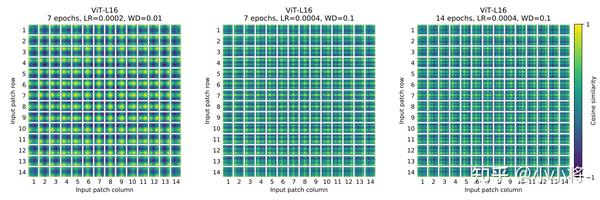def resize_pos_embed(posemb, posemb_new):
_logger.info('Resized position embedding: %s to %s', posemb.shape, posemb_new.shape)
ntok_new = posemb_new.shape
# 除去class token的pos_embed
posemb_tok, posemb_grid = posemb[:, :1], posemb[0, 1:]
ntok_new -= 1
gs_old = int(math.sqrt(len(posemb_grid)))
gs_new = int(math.sqrt(ntok_new))
_logger.info('Position embedding grid-size from %s to %s', gs_old, gs_new)
# 把pos_embed变换到2-D维度再进行插值
posemb_grid = posemb_grid.reshape(1, gs_old, gs_old, -1).permute(0, 3, 1, 2)
posemb_grid = F.interpolate(posemb_grid, size=(gs_new, gs_new), mode='bilinear')
posemb_grid = posemb_grid.permute(0, 2, 3, 1).reshape(1, gs_new * gs_new, -1)
posemb = torch.cat([posemb_tok, posemb_grid], dim=1)
return posemb

## Class Token

# 随机初始化
self.cls_token = nn.Parameter(torch.zeros(1, 1, embed_dim))

self.head = nn.Linear(self.num_features, num_classes) if num_classes > 0 else nn.Identity()

# 具体forward过程
B = x.shape
x = self.patch_embed(x)
cls_tokens = self.cls_token.expand(B, -1, -1)  # stole cls_tokens impl from Phil Wang, thanks
x = torch.cat((cls_tokens, x), dim=1)
x = x + self.pos_embed

## Transformer Encoder

transformer最核心的操作就是self-attention，其实attention机制很早就在NLP和CV领域应用了，比如带有attention机制的seq2seq模型，但是transformer完全摒弃RNN或LSTM结构，直接采用attention机制反而取得了更好的效果：attention is all you need！简单来说，attention就是根据当前查询对输入信息赋予不同的权重来聚合信息，从操作上看就是一种“加权平均”。attention中共有3个概念：query, key和value，其中key和value是成对的，对于一个给定的query向量$q\in \mathbb{R}^{d}$，通过内积计算来匹配k个key向量（维度也是d，堆积起来即矩阵$K\in \mathbb{R}^{k\times d}$），得到的内积通过softmax来归一化得到k个权重，那么对于query其attention的输出就是k个key向量对应的value向量（即矩阵$V\in \mathbb{R}^{k\times d}$）的加权平均值。对于一系列的N个query（即矩阵$Q\in \mathbb{R}^{N\times d}$），可以通过矩阵计算它们的attention输出：

$$Attention(Q, K, V) = Softmax(\frac{QK^T}{\sqrt{d_k}})V \$$

$$MSA(X) = Concat(head_1, ..., head_h) W^O, head_i=SA(XW_i^Q, XW_i^K, XW_i^V) \$$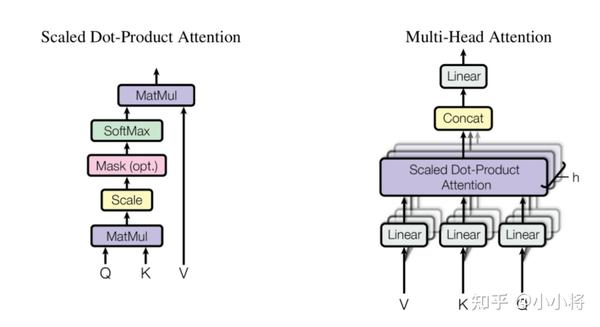MSA的计算量是和$N^2$成正相关的，所以ViT的输入是patch embeddings，而不是pixel embeddings，这有计算量上的考虑。在实现上，MSA是可以并行计算各个head的，具体代码如下：

class Attention(nn.Module):
def __init__(self, dim, num_heads=8, qkv_bias=False, qk_scale=None, attn_drop=0., proj_drop=0.):
super().__init__()

self.scale = qk_scale or head_dim ** -0.5

self.qkv = nn.Linear(dim, dim * 3, bias=qkv_bias)
self.attn_drop = nn.Dropout(attn_drop)
self.proj = nn.Linear(dim, dim)
# 这里包含了dropout
self.proj_drop = nn.Dropout(proj_drop)

def forward(self, x):
B, N, C = x.shape
qkv = self.qkv(x).reshape(B, N, 3, self.num_heads, C // self.num_heads).permute(2, 0, 3, 1, 4)
q, k, v = qkv, qkv, qkv   # make torchscript happy (cannot use tensor as tuple)

attn = (q @ k.transpose(-2, -1)) * self.scale
attn = attn.softmax(dim=-1)
attn = self.attn_drop(attn)

x = (attn @ v).transpose(1, 2).reshape(B, N, C)
x = self.proj(x)
x = self.proj_drop(x)
return x

class Mlp(nn.Module):
def __init__(self, in_features, hidden_features=None, out_features=None, act_layer=nn.GELU, drop=0.):
super().__init__()
out_features = out_features or in_features
hidden_features = hidden_features or in_features
self.fc1 = nn.Linear(in_features, hidden_features)
self.act = act_layer()
self.fc2 = nn.Linear(hidden_features, out_features)
self.drop = nn.Dropout(drop)

def forward(self, x):
x = self.fc1(x)
x = self.act(x)
x = self.drop(x)
x = self.fc2(x)
x = self.drop(x)
return x

class Block(nn.Module):

def __init__(self, dim, num_heads, mlp_ratio=4., qkv_bias=False, qk_scale=None, drop=0., attn_drop=0.,
drop_path=0., act_layer=nn.GELU, norm_layer=nn.LayerNorm):
super().__init__()
self.norm1 = norm_layer(dim)
self.attn = Attention(
# NOTE: drop path for stochastic depth, we shall see if this is better than dropout here
self.drop_path = DropPath(drop_path) if drop_path > 0. else nn.Identity()
self.norm2 = norm_layer(dim)
mlp_hidden_dim = int(dim * mlp_ratio)
self.mlp = Mlp(in_features=dim, hidden_features=mlp_hidden_dim, act_layer=act_layer, drop=drop)

def forward(self, x):
x = x + self.drop_path(self.attn(self.norm1(x)))
x = x + self.drop_path(self.mlp(self.norm2(x)))
return x

## ViT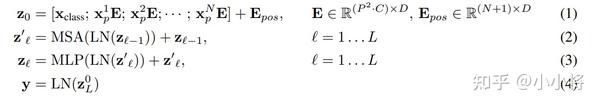ViT模型的超参数主要包括以下，这些超参数直接影响模型参数以及计算量：

1. Layers：block的数量；
2. Hidden size D：隐含层特征，D在各个block是一直不变的；
3. MLP size：一般设置为4D大小；
5. Patch size：模型输入的patch size，ViT中共有两个设置：14x14和16x16，这个只影响计算量；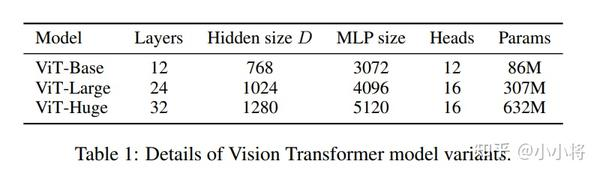### 模型效果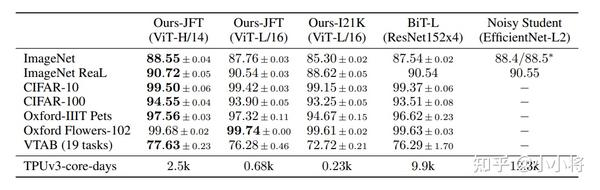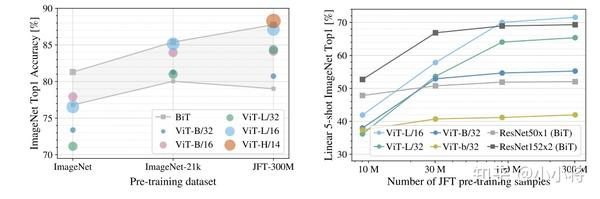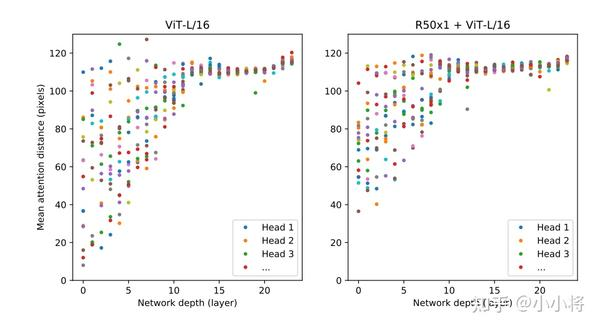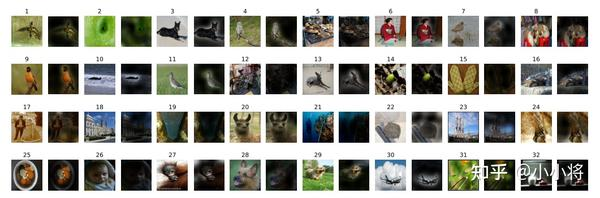• [DeiT] Training data-efficient image transformers \& distillation through attention
• [T2T-ViT] Tokens-to-Token ViT: Training Vision Transformers from Scratch on ImageNet
• [CPVT] Do We Really Need Explicit Position Encodings for Vision Transformers\?
• [PVT] Pyramid Vision Transformer: A Versatile Backbone for Dense Prediction without Convolutions
• [TNT] Transformer in Transformer

### 参考

•0
•0
•4118收藏

• 62

关注
• 220

获赞
• 15

精选文章

• 目标跟踪

• 目标跟踪分享

• 280
• 307
• 234
• 283
• 372